Next: Uniform circular motion Up: Oscillatory motion Previous: The simple pendulum

## The compound pendulum

Consider an extended body of mass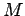with a hole drilled though it. Suppose that the body is suspended from a fixed peg, which passes through the hole, such that it is free to swing from side to side, as shown in Fig. 98. This setup is known as a compound pendulum.Let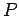be the pivot point, and let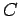be the body's centre of mass, which is located a distancefrom the pivot. Letbe the angle subtended between the downward vertical (which passes through point) and the line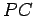. The equilibrium state of the compound pendulum corresponds to the case in which the centre of mass lies vertically below the pivot point: i.e.,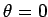. See Sect. 10.3. The angular equation of motion of the pendulum is simply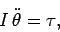(529)

where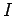is the moment of inertia of the body about the pivot point, and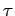is the torque. Using similar arguments to those employed for the case of the simple pendulum (recalling that all the weight of the pendulum acts at its centre of mass), we can write(530)

Note that the reaction,, at the peg does not contribute to the torque, since its line of action passes through the pivot point. Combining the previous two equations, we obtain the following angular equation of motion of the pendulum: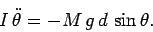(531)

Finally, adopting the small angle approximation,, we arrive at the simple harmonic equation: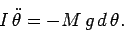(532)

It is clear, by analogy with our previous solutions of such equations, that the angular frequency of small amplitude oscillations of a compound pendulum is given by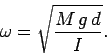(533)

It is helpful to define the length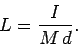(534)

Equation (533) reduces to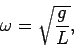(535)

which is identical in form to the corresponding expression for a simple pendulum. We conclude that a compound pendulum behaves like a simple pendulum with effective length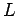.Next: Uniform circular motion Up: Oscillatory motion Previous: The simple pendulum
Richard Fitzpatrick 2006-02-02